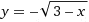# Is this considered as a function?such that x is real number and x =< 3

arildno
Homework Helper
Gold Member
Dearly Missed
Yes.
To each valid input of x, there is only one associated y-value.

I.e, "y" is a function of x.

Actually, it is an equation, not a function. This equation can be used to define a function by someone who knows what he is doing.

chiro
View attachment 35474 such that x is real number and x =< 3

For future reference, one easy test to check if something is a function is to do a vertical line test. Basically what you do is if you find a point where a vertical line crosses two or more points it is not an explicit function. To make it an explicit function you need to restrict the domain.

So as an example x^2 + y^2 = 1 is the unit circle, but is not a function unless you restrict the domain. You can draw a vertical line it will always intersect two points unless your y value is zero.

For clarification, we're talking about "one to one" functions.

HallsofIvy
Homework Helper
Actually, it is an equation, not a function. This equation can be used to define a function by someone who knows what he is doing.
No, "$y= \sqrt{3- x}$" is an equation but the additional "such that x is a real number and $x\le 3$" states a domain and makes this a function.

For clarification, we're talking about "one to one" functions.
Where did you get that idea? The original function happens to be one to one but that was irrelevant to the question and to the responses.

Where did you get that idea? The original function happens to be one to one but that was irrelevant to the question and to the responses.

Right, true. Yes, ok. Fine, but functions don't by definition need to be one-to-one. That's just an injective ("one-to-one") function. Restricting the domain to pass the vertical line test doesn't need to happen to define it as a function...just a one-to-one function.

I thought if followed and might be useful for the poster to know about the broader categories of functions than just the injective type. A function is many things. An injective function passes the vertical line test, if you like. No disrespect intended.

Restricting the domain to pass the vertical line test doesn't need to happen to define it as a function...just a one-to-one function.

No, every function must pass the vertical line test. It is the horizontal line test that is used to determine the injective functions.

Or am I messing up horizontal and vertical again?

I mean function like "mapping elements from a set A to a set B." (Rudin, 3rd ed.) You know? No bijectivity required.

no, a function must pass the vertical line test. A one-one function passes a similar "horizontal line test". This is by definition
If the vertical line test fails, you can call it some sort of " general mapping" from a set A to a set B, but it may not be well-defined.
For micromass, | <-- vertical __ <-- horizontal, think of the horizon :P

Yes, the base definition for a function is that for every element of x, there is a unique y.

you can call it some sort of " general mapping" from a set A to a set B, but it may not be well-defined.

It's the "general math" forum! The answer should be general!

;-)

Last edited:
It's the "general math" forum! The answer should be general!

;-)

Well, a function is *not* some sort of "general" mapping, and that is what the poster specifically asked for. Furthermore, a non-function mapping may not be well-defined, and thus be non sensical: suppose f ( x ) = y and f ( x ) = t, where y != t. Then f(x) = y != t??

A function is the most "general mapping" that is worth talking about, a non-function mapping can just be nonsense.

Oops! You win. "Relation." Apparently I forgot "relation."